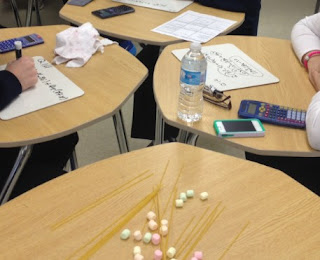## Friday, 18 December 2015

### Number Sense and Algebra Tower ChallengeThis is a review activity on number sense and algebra for grade 9 academic where students answer questions and are rewarded with building materials for each correct answer. The building materials (spagetti & marshmallows) are then used with the goal being the creation of tallest tower. This is based originally on a TIPS activity on quadratics for MBF3C (Unit 3, Day 6).  We have a similar activity for grade 9 applied that can be found here

MPM 1D
• substitute into and evaluate algebraic expressions involving exponents
• describe the relationship between the algebraic and geometric representations of a single-variable term up to degree three [i.e., length, which is one dimensional, can be represented by x; area, which is two dimensional, can be represented by (x)(x) or x2; volume, which is three dimensional,can be represented by (x)(x)(x), (x2)(x),or x3]
• derive, through the investigation and examination of patterns, the exponent rules for multiplying and dividing monomials, and apply these rules in expressions involving one and two variables with positive exponents
• extend the multiplication rule to derive and understand the power of a power rule, and apply it to simplify expressions involving one and two variables with positive exponents.
• relate their understanding of inverse operations to squaring and taking the square root, and apply inverse operations to simplify expressions and solve equations
• add and subtract polynomials with up to two variables
• multiply a polynomial by a monomial involving the same variable
• expand and simplify polynomial expressions involving one variable
• solve first-degree equations, including equations with fractional coefficients, using a variety of tools
• rearrange formulas involving variables in the first degree, with and without substitution
• solve problems that can be modelled with first-degree equations, and compare algebraic methods to other solution methods
• 1 bag of spaghetti and 1-2 bags of small marshmallows (or 1 box of straws and 1-inch pieces of tape)
• a question sheet for each student
• Optional - a whiteboard for each student to work out their solutions
• Optional - prize for the group with the tallest tower1. Place students in groups (ideally no bigger than 3 per group)
2. Hand out question sheets (and optional whiteboards) to each student.
3. Have students answer questions from their sheet in any order they want. For every correct answer they will get some building materials (eg: 2 spagetti & 3 marshmallows, the amount of each reward is indicated on the student question sheet ). The harder the question the more materials they will get. Eventually the building materials will be used to create a tower with the goal to create the tallest free standing tower.
4. Students work in groups to answer the questions and bring their solutions up to you to be checked. Only one member from each group can come up at a time. Each group can answer each question only once. To keep track of this, use the teacher answer sheet to check off which questions each group has answered as they come up.
5. Leave about 20 min at the end of the class for students to create their towers (students can no longer answer questions)
6. Take lots of pictures and celebrate the group with the tallest free standing tower